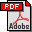Kahden merkkijonon pisimmän yhteisen alijonon ongelma ja sen ratkaiseminen

Lasse Bergroth, Kahden merkkijonon pisimmän yhteisen alijonon ongelma ja sen ratkaiseminen. TUCS Dissertations 146. University of Turku, 2012.

Abstract:

The topic of this thesis belongs to string algorithmics. A string denoted by S is a common subsequence of strings X[1..m] and Y[1..n], if it can be extracted by deleting arbitrarily 0..m symbols from X and 0..n symbols from Y. If no common subsequence of X and Y is longer than S, it is determined that S is the longest common subsequence
(abbr. LCS) of X and Y. In this work, solving the LCS problem for two strings will be concerned, but the problem can also be generalized for several strings.

LCS problem has applications not only in computer science but also in research areas of bioinformatics. To the best known applications belong text and image
compression, version maintenance of files, pattern recognition and comparison research of DNA and protein structures. Solving the problem is difficult, because the algorithms depend on several parameters of the input strings. To those belong, for instance, length
of the input strings, size of the input alphabet, character distribution of the inputs, proportion of LCS in the shorter input string and amount of matching symbol pairs.
Thus it is difficult to develop an algorithm which could run effectively for all problem instances.

The thesis should on the one hand be regarded as a handbook, where, after the description of basic concepts, already earlier developed exact LCS algorithms will be
introduced. They are considered in groups which are classified according to the processing model of the algorithm: one row, contour or one diagonal at a time or multidirectedly. In addition, heuristic methods for calculating an upper or a lower bound for LCS will be represented. The results calculated by those methods can be utilized either as such or in order to steer the processing of an exact algorithm. This section is based on
articles published by our research group. Exact LCS algorithms enforced with heuristic preprocessing are introduced for the first time in those articles.

On the other hand, the work contains quite a comprehensive section of empirical research, which aims at intensifying the running time and reduction of the memory consumption of existing exact algorithms. This target was tried to be reached in terms of programming techniques by introducing well-supporting data structures for the processing model of the algorithms, and by restricting fruitless calculation performed by the algorithms by improving their capability to detect intermediate results obtained once during the running time and to utilize them.

As conclusions of the thesis, it can be generally mentioned that the heuristic preprocessing almost systematically reduces the running time and especially the memory need of exact LCS algorithms. Furthermore, the data structure of the algorithms has a crucial influence on the efficiency of the calculation: the more local the search and update operations are, the more efficient is the calculation of the algorithm.

Files:Full publication in PDF-format

BibTeX entry:

@PHDTHESIS{phdBergroth_Lasse12a,
title = {Kahden merkkijonon pisimmän yhteisen alijonon ongelma ja sen ratkaiseminen},
author = {Bergroth, Lasse},
number = {146},
series = {TUCS Dissertations},
school = {University of Turku},
year = {2012},
keywords = {string algorithms, longest common subsequence, LCS heuristics},
ISBN = {978-952-12-2756-1},
}

Belongs to TUCS Research Unit(s): Algorithmics and Computational Intelligence Group (ACI)

Edit publication